﻿ 基于改进A-Star算法的隐身无人机快速突防航路规划Download PDF文章快速检索 高级检索

1. 南昌航空大学 信息工程学院, 南昌 330063;
2. 北京航空航天大学 可靠性与系统工程学院, 北京 100083

Fast penetration path planning for stealth UAV based on improved A-Star algorithm
ZHANG Zhe1, WU Jian1,2, DAI Jiyang1, YING Jin1, HE Cheng1
1. School of Information Engineering, Nanchang Hangkong University, Nanchang 330063, China;
2. School of Reliability and System Engineering, Beihang University, Beijing 100083, China
Abstract: Aiming to solve the survival and penetration problems of the stealth Unmanned Aerial Vehicle (UAV) under high-definition netted radar defense systems in modern warfare, this paper proposes a stealth UAV theater penetration path planning technology based on an improved A-Star algorithm. Firstly, the penetration process of the stealth UAV is analyzed and modeled, and the kinematics model of the stealth UAV, the dynamic radar cross section characteristics and the calculation model of the netted radar detection probability are established. Then, in view of the shortcomings of traditional algorithms in solving the problem of stealth penetration and taking into full consideration of the requirements of rapidity and safety in the planned route, an improved A-Star algorithm is designed. The multi-layer variable step size search strategy and the attitude angle information of UAVs are introduced into the algorithm. Further combined with the rank K fusion criterion, this algorithm can judge the feasibility of the new track point by the detection probability of the netted radar system of the stealth UAV on each track. The simulation results show that the improved A-Star algorithm can quickly generate better penetration routes in the combat area under complex netted radar systems, exhibiting certain application value.
Keywords: unmanned aerial vehicles    route planning    netted radar    A-Star algorithm    radar cross sections

1 隐身无人机突防过程建模与分析

1.1 隐身无人机运动学模型

 $\left\{ {\begin{array}{*{20}{l}} {\dot x = v{\kern 1pt} {\kern 1pt} {\rm{cos}}{\kern 1pt} {\kern 1pt} {\kern 1pt} \varphi }\\ {\dot y = v{\kern 1pt} {\kern 1pt} {\rm{sin}}{\kern 1pt} {\kern 1pt} {\kern 1pt} \varphi }\\ {\dot \varphi = \frac{{g{\kern 1pt} {\rm{tan}}{\kern 1pt} {\kern 1pt} {\kern 1pt} \theta }}{v}} \end{array}} \right.$ （1）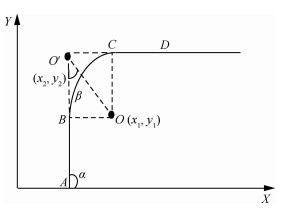图 1 隐身无人机的运动状态 Fig. 1 Movement state of stealth UAV

 $\left[ {\begin{array}{*{20}{l}} {{x_1}}\\ {{y_1}} \end{array}} \right] = \left[ {\begin{array}{*{20}{l}} {{x_2}}\\ {{y_2}} \end{array}} \right] + \frac{{{l_{A{O^\prime }}}}}{{b{\kern 1pt} {\kern 1pt} {\rm{cos}}{\kern 1pt} {\kern 1pt} \beta }}\left[ {\begin{array}{*{20}{c}} {{\rm{cos}}(\pi - \alpha - \beta )}\\ { - {\rm{sin}}(\pi - \alpha - \beta )} \end{array}} \right]$ （2）

1) 最大负载飞行模式。无人机在转弯时保持较大的滚转角，这样可以保证转弯半径r最小，转弯所需时间最少，暴露于雷达中的时间相对较短。

2) 最小滚转角飞行模式。假设图 1中线段AO′BO′是无人机飞行中相邻的2个航线，当B点为线段AO′的中点，C点为线段O′D的中点时，无人机能够以最大转弯半径保持转弯飞行，这样便可以在飞行过程中始终保持相对较小的滚转角θ，大大降低了雷达系统的探测概率。

 $\frac{r}{{{\rm{sin}}{\kern 1pt} \beta }} = \frac{{{l_{A{O^\prime }}}}}{{b{\kern 1pt} {\kern 1pt} {\rm{cos}}{\kern 1pt} {\kern 1pt} \beta }}$ （3）

rminrmax分别表示无人机的最小转弯半径及最大转弯半径，由式(3)计算可得无人机飞行过程中的最大转弯半径为

 ${r_{{\rm{max}}}} = \frac{{{l_{A{O^\prime }}}{\rm{tan}}{\kern 1pt} \beta }}{b}$ （4）

θmaxθmin分别表示无人机飞行时的最大滚转角和最小滚转角，分析式(1)中无人机的运动学模型及图 1中的航线几何关系，可以计算得到：

 $\left\{ {\begin{array}{*{20}{l}} {{\theta _{{\rm{max}}}} = {\rm{arctan}}\frac{{{v^2}}}{{g{r_{{\rm{min}}}}}}}\\ {{\theta _{{\rm{min}}}} = {\rm{arctan}}\frac{{{v^2}}}{{g{r_{{\rm{max}}}}}} = \frac{{b{v^2}}}{{g{l_{A{O^\prime }}}{\kern 1pt} {\rm{tan}}{\kern 1pt} \beta }}} \end{array}} \right.$ （5）

1.2 动态RCS特性

1.3 雷达的探测概率

1.3.1 单雷达系统的探测概率

 $\left\{ {\begin{array}{*{20}{l}} {P = \frac{1}{T}\int\limits_{t - T}^t {{P_t}} {\rm{d}}t}\\ {{P_t} = \frac{1}{{1 + {{\left( {\frac{{{c_2}R_i^4}}{\sigma }} \right)}^{{c_1}}}}}} \end{array}} \right.$ （6）

 $P = 1 - \prod\limits_{i = 1}^s {(1 - {p_i})}$ （7）

1.3.2 组网雷达系统的探测概率

 ${K_0} = 1.5\sqrt M$ （8）

K融合规则判决流程如图 2所示。每部雷达根据自身对隐身无人机的探测做出局部判断，判决结果非0即1，取决于局部门限检测目标是否存在。由二元假设为H0H1H0表示目标不出现，H1表示目标出现。因此第j个雷达的决策值dj(j=1, 2, …, M)可以表示为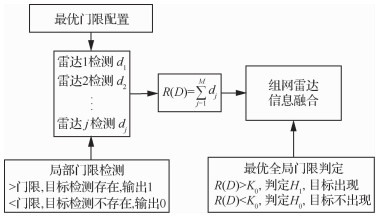图 2 秩K融合规则判决流程 Fig. 2 Rank K fusion rule decision process
 ${d_j} = \left\{ {\begin{array}{*{20}{l}} 0&{{\rm{ 若第 }}j{\rm{ 个雷达判定}}{H_0}}\\ 1&{{\rm{ 若第 }}j{\rm{ 个雷达判定 }}{H_1}} \end{array}} \right.$ （9）

 $R(D) = \left\{ {\begin{array}{*{20}{l}} 1&{\sum\limits_{j = 1}^M {{d_j}} \ge {K_0}}\\ 0&{\sum\limits_{j = 1}^M {{d_j}} < {K_0}} \end{array}} \right.$ （10）

 ${P_D} = \sum\limits_D {\left[ {R(D)\prod\limits_{{d_j} \in {S_1}} {{P_{{d_j}}}} \prod\limits_{{d_j} \in {S_0}} {(1 - {P_{{d_j}}})} } \right]}$ （11）

 ${P_{\rm{F}}} = \sum\limits_D {\left[ {R(D)\prod\limits_{{d_j} \in {S_1}} {{P_{{{\rm{f}}_j}}}} \prod\limits_{{d_j} \in {S_0}} {(1 - {P_{{{\rm{f}}_j}}})} } \right]}$ （12）
2 突防航迹规划算法

A-Star算法作为一种启发式搜索算法，广泛应用于各类智能体的路径规划问题中[19-21]。本文引入A-Star算法用于隐身无人机的战区突防航路规划，从隐身无人机航路搜索的快速性和安全性两方面入手进行深入分析。

2.1 算法描述

 $f(n) = g(n) + h(n)$ （13）

2.2 改进A-Star算法 2.2.1 无人机姿态角信息融合

 ${\theta _n} = {\rm{co}}{{\rm{s}}^{ - 1}}\frac{1}{{\sqrt {{{[({\varphi _n} - {\varphi _{\rm{s}}}){v^2}/\eta g]}^2} + 1} }}$ （14）

 $|{\varphi _n} - {\varphi _{\rm{s}}}| \le \frac{{|{x_n} - {x_{\rm{s}}}|}}{{{{\dot \varphi }_{{\rm{max}}}}}}$ （15）

2.2.2 多层变步长策略

 $f(n) = g(n) + \omega h(n)$ （16）

 $\left\{ {\begin{array}{*{20}{l}} {{x_{k + 1}} = {x_k} + {L_j}{\kern 1pt} {\rm{cos}}{\kern 1pt} {\kern 1pt} {\phi _i}}\\ {{y_{k + 1}} = {y_k} + {L_j}{\kern 1pt} {\rm{sin}}{\kern 1pt} {\kern 1pt} {\phi _i}} \end{array}} \right.$ （17）

 $\left\{ {\begin{array}{*{20}{l}} {{\phi _i} = {\phi _0}i = \frac{{{\phi _{{\rm{max}}}}}}{n}i}\\ {{L_j} = {L_0}j = \frac{L}{m}j} \end{array}} \right.$ （18）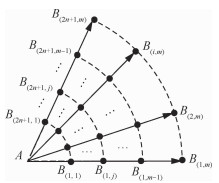图 3 变步长搜索航迹点拓展过程 Fig. 3 Variable step size search track point expansion process
2.2.3 新航迹点的可行性判定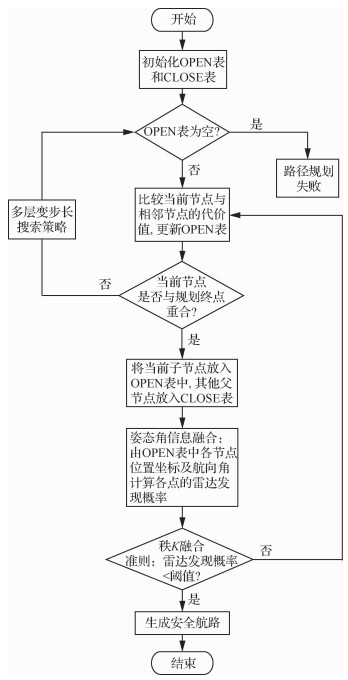图 4 改进A-Star算法的流程图 Fig. 4 Flowchart of improved A-Star algorithm
3 仿真实验分析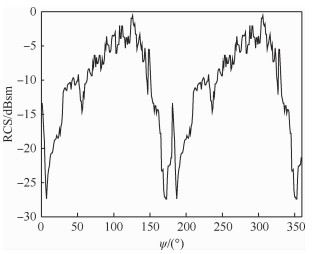图 5 某型隐身无人机的全向RCS曲线(S波段HH极化) Fig. 5 Omnidirectional RCS curve of a stealth UAV(S-band HH polarization)
3.1 单雷达系统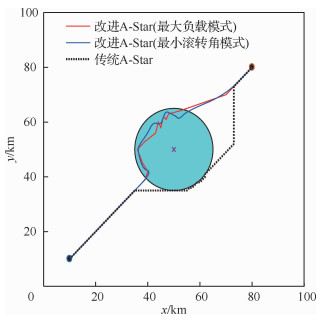图 6 单雷达系统下的突防仿真结果 Fig. 6 Penetration simulation results under single radar system

 算法 飞行距离/km 高发现概率均值 计算耗时/s 改进A-Star(最大负载模式) 108.92 0.412 5.03 改进A-Star(最小滚转角模式) 110.37 0.403 5.14 传统A-Star 111.88 0.431 8.49

3.2 组网雷达系统

3.2.1 3雷达系统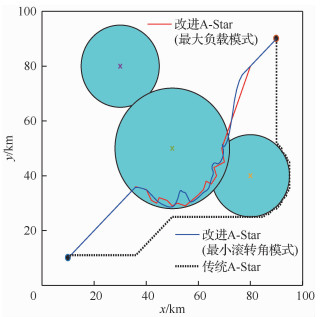图 7 3雷达系统下的突防仿真结果 Fig. 7 Penetration simulation results under three radars system

 算法 飞行距离/km 高发现概率均值 计算耗时/s 改进A-Star(最大负载模式) 138.43 0.433 5.94 改进A-Star(最小滚转角模式) 147.49 0.426 6.16 传统A-Star 153.60 0.454 7.19

3.2.2 5雷达系统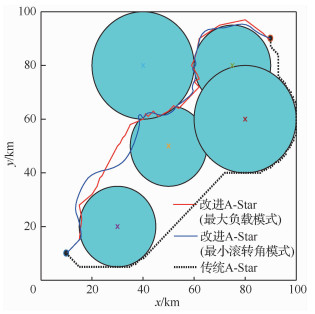图 8 5雷达系统下的突防仿真结果 Fig. 8 Penetration simulation results under five radars system

 算法 飞行距离/km 高发现概率均值 计算耗时/s 改进A-Star(最大负载模式) 146.03 0.463 8.43 改进A-Star(最小滚转角模式) 154.27 0.442 8.97 传统A-Star 151.41 0.513 11.56
3.2.3 10雷达系统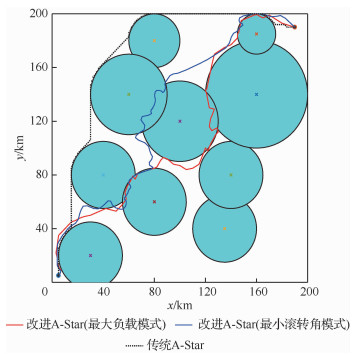图 9 10雷达系统下的突防仿真结果 Fig. 9 Penetration simulation results under ten radars system

 算法 飞行距离/km 高发现概率均值 计算耗时/s 改进A-Star(最大负载模式) 353.48 0.474 17.51 改进A-Star(最小滚转角模式) 383.65 0.451 19.36 传统A-Star 345.48 0.692 31.33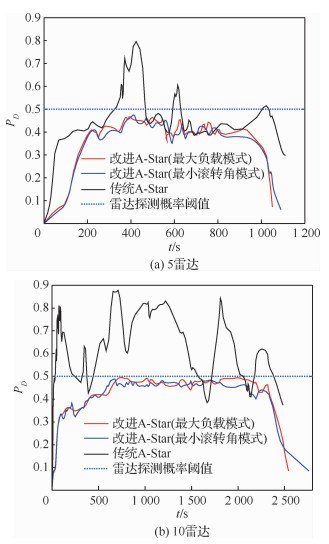图 10 典型威胁环境下不同算法的突防威胁概率 Fig. 10 Penetration threat probability of different algorithms in typical threat environment

4 结论

1) 针对复杂的组网雷达系统下隐身无人机实现快速和安全的突防问题，提出了一种改进A-Star算法的隐身无人机战区突防航路规划方法，并将其与传统A-Star算法进行比较，仿真结果表明改进算法优于传统算法。

2) 所设计的改进A-Star算法在对航迹节点的搜索过程中引入了多层变步长搜索策略和无人机的姿态角信息，加快了航路搜索的效率，体现了隐身无人机动态RCS的变化特性，为复杂战场环境中的隐身无人机突防提供了一定的军事应用价值。

  ZEITZ III F H. UCAV path planning in the presenceof radar-guided surface-to-air missile threats[D]. Michigan: University of Michigan, 2005.  LI Y, WU Z, HUANG P L. A new method for analyzing integrated stealth ability of penetration aircraft[J]. Chinese Journal of Aeronautics, 2010, 23(2): 187-193. Click to display the text  ALVES M A, PORT R J, REZENDE M C. Simulations of the radar cross section of a stealth aircraft[C]//2007 SBMO/IEEE MTT-S International Microwave and Optoelectronics Conference. Piscataway: IEEE Press, 2007: 409-412.  LI Y, HUANG J, HONG S, et al. A new assessment method for the comprehensive stealth performance of penetration aircrafts[J]. Aerospace Science and Technology, 2011, 15(7): 511-518. Click to display the text  陈世春, 黄沛霖, 姬金祖. 典型隐身飞机的RCS起伏统计特性[J]. 航空学报, 2014, 35(12): 3304-3314. CHEN S C, HUANG P L, JI J Z. Radar cross section fluctuation characteristics of typical stealth aircraft[J]. Acta Aeronautica et Astronautica Sinica, 2014, 35(12): 3304-3314. (in Chinese) Cited By in Cnki (20) | Click to display the text  AMR M, JING R, ALHOSSEIN M., et al. Optimal path planning for unmanned ground vehicles using potential field method and optimal control method[J]. International Journal of Vehicle Performance, 2018, 4(1): 1-14. Click to display the text  HE P, DAI S. Stealth coverage multi-path corridors planning for UAV fleet[C]//Proceedings 2013 Internati-onal Conference on Mechatronic Sciences, Electric Engineering and Computer (MEC). Piscataway: IEEE Press, 2013: 2922-2926.  INANC T, MUEZZINOGLU M K, MISOVEC K, et al. Framework for low-observable trajectory generation in presence of multiple radars[J]. Journal of Guidance, Control, and Dynamics, 2008, 31(6): 1740-1749. Click to display the text  WU P P Y, CAMPBELL D, MERZ T. Multi-objective four-dimensional vehicle motion planning in large dynamic environments[J]. IEEE Transactions on Systems, Man, and Cybernetics, Part B (Cybernetics), 2010, 41(3): 621-634. Click to display the text  晏青, 熊峻江, 游思明. 基于动态RCS的无人机航迹实时规划[J]. 北京航空航天大学学报, 2011, 37(9): 1115-1121. YAN Q, XIONG J J, YOU S M. Real-time programming method for flight path of unmanned vehiclebased on dynamic RCS[J]. Journal of Beijing University of Aeronautics and Astronautics, 2011, 37(9): 1115-1121. (in Chinese) Cited By in Cnki | Click to display the text  KABAMBA P T, MEERKOV S M, ZEITZ F H. Optimal path planning for unmanned combat aerial vehicles to defeat radar tracking[J]. Journal of Guidance, Control, and Dynamics, 2006, 29(2): 279-288. Click to display the text  史伟强, 徐乐, 史小卫. 基于完备对数正态分布模型的隐形飞行器动态RCS统计特性研究[J]. 电子与信息学报, 2013(9): 93-97. SHI W Q, XU L, SHI X W. Dynamic RCS statistic characterization of stealth aircraft using complete lognormal distribution[J]. Journal of Electronic & Information Technology, 2013(9): 93-97. (in Chinese) Cited By in Cnki (13) | Click to display the text  丁晓东, 刘毅, 李为民. 基于动态RCS的无人机航迹实时规划方法研究[J]. 系统工程与电子技术, 2008, 30(5): 868-871. DING X D, LIU Y, LI W M. Dynamic RCS and real-time based analysis of method of UAV route planning[J]. Systems Engineering and Electroncis, 2008, 30(5): 868-871. (in Chinese) Cited By in Cnki | Click to display the text  田阔, 符小卫, 高晓光. 威胁联网下无人机路径在线规划[J]. 西北工业大学学报, 2011, 29(3): 367-373. TIAN K, FU X W, GAO X G. Exploring further UAV on-line path planning in the presence of threat netting[J]. Journal of Northwestern Polytechnical University, 2011, 29(3): 367-373. (in Chinese) Cited By in Cnki (23) | Click to display the text  GRANT R. The radar game:Understanding stealth and aircraft survivability[M]. Arlington: IRIS Independent Research, 1998.  SCHWARTZ M. A coincidence procedure for signal detection[J]. IRE Transactions on Information Theory, 1956, 2(4): 135-139.  CAO Y, LONG T, WANG Z, et al. Aircraft route planning for stealth penetration based on sparse A* search[C]//201729th Chinese Control and Decision Conference (CCDC). Piscataway: IEEE Press, 2017: 5380-5385.  莫松, 黄俊, 郑征, 等. 基于改进快速扩展随机树方法的隐身无人机突防航迹规划[J]. 控制理论与应用, 2014, 31(3): 375-385. MO S, HUANG J, ZHENG Z, et al. Stealth penetration path planning for stealth ummanned aerial vehicle based on improved rapidly exploring random tree[J]. Control Theory & Applications, 2014, 31(3): 375-385. (in Chinese) Cited By in Cnki (3) | Click to display the text  DUCHO F, BABINEC A, KAJAN M, et al. Path planning with modified a star algorithm for a mobile robot[J]. Procedia Engineering, 2014, 96: 59-69. Click to display the text  CHENG L, LIU C, YAN B. Improved hierarchical Astar algorithm for optimal parking path planning of the large parking lot[C]//2014 IEEE International Conference on Information and Automation (ICIA). Piscataway: IEEE Press, 2014: 695-698.  CHANG W Y, HSIAO F B, SHEU D. Two-point flightpath planning using a fast graph-search algorithm[J]. Journal of Aerospace Computing, Information, and Communication, 2006, 3(9): 453-488. Click to display the text  GAO X, REN J, CHEN D. Developing an effective algorithm for dynamic UAV path planning with incomplete threat information[J]. Proceedings of the Institution of Mechanical Engineers, Part G:Journal of Aerospace Engineering, 2012, 226(4): 413-421. Click to display the text  WU P P Y, CAMPBELL D, MERZ T. Multi-objective four-dimensional vehicle motion planning in large dynamic environments[J]. IEEE Transactions on Systems, Man, and Cybernetics, Part B (Cybernetics), 2010, 41(3): 621-634. Click to display the text
http://dx.doi.org/10.7527/S1000-6893.2020.23692

0

#### 文章信息

ZHANG Zhe, WU Jian, DAI Jiyang, YING Jin, HE Cheng

Fast penetration path planning for stealth UAV based on improved A-Star algorithm

Acta Aeronautica et Astronautica Sinica, 2020, 41(7): 323692.
http://dx.doi.org/10.7527/S1000-6893.2020.23692